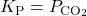Chapter 13. Chemical Equilibrium

# The Equilibrium Constant

Learning Objectives

1. Explain the importance of the equilibrium constant.
2. Construct an equilibrium constant expression for a chemical reaction.

In the mid 1860s, Norwegian scientists C. M. Guldberg and P. Waage noted a peculiar relationship between the amounts of reactants and products in an equilibrium. No matter how many reactants they started with, a certain ratio of reactants and products was achieved at equilibrium. Today, we call this observation the law of mass action. It relates the amounts of reactants and products at equilibrium for a chemical reaction. For a general chemical reaction occurring in solution,

aA + bB ⇄ cC + dD

the equilibrium constant, also known as Keq, is defined by the following expression: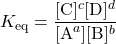where [A] is the molar concentration of species A at equilibrium, and so forth. The coefficients a, b, c, and d in the chemical equation become exponents in the expression for Keq. The Keq is a characteristic numerical value for a given reaction at a given temperature; that is, each chemical reaction has its own characteristic Keq. The concentration of each reactant and product in a chemical reaction at equilibrium is related; the concentrations cannot be random values, but they depend on each other. The numerator of the expression for Keq has the concentrations of every product (however many products there are), while the denominator of the expression for Keq has the concentrations of every reactant, leading to the common products over reactants definition for the Keq.

Let us consider a simple example. Suppose we have this equilibrium:

A ⇄ B

There is one reactant, one product, and the coefficients on each are just 1 (assumed, not written). The Keq expression for this equilibrium is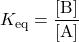(Exponents of 1 on each concentration are understood.)

Suppose the numerical value of Keq for this chemical reaction is 2.0. If [B] = 4.0 M, then [A] must equal 2.0 M so that the value of the fraction equals 2.0: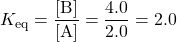By convention, the units are understood to be M and are omitted from the Keq expression. Suppose [B] were 6.0 M. For the Keq value to remain constant (it is, after all, called the equilibrium constant), then [A] would have to be 3.0 M at equilibrium: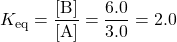If [A] were not equal to 3.0 M, the reaction would not be at equilibrium, and a net reaction would occur until that ratio was indeed 2.0. At that point, the reaction is at equilibrium, and any net change would cease. (Recall, however, that the forward and reverse reactions do not stop because chemical equilibrium is dynamic.)

The issue is the same with more complex expressions for the Keq; only the mathematics becomes more complex. Generally speaking, given a value for the Keq and all but one concentration at equilibrium, the missing concentration can be calculated.

Example 13.2

Given the following reaction:

H2 + I2 ⇄ 2HI

If the equilibrium [HI] is 0.75 M and the equilibrium [H2] is 0.20 M, what is the equilibrium [I2] if the Keq is 0.40?

Solution
We start by writing the Keq expression. Using the products over reactants approach, the Keq expression is as follows: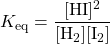Note that [HI] is squared because of the coefficient 2 in the balanced chemical equation. Substituting for the equilibrium [H2] and [HI] and for the given value of Keq: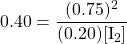To solve for [I2], we have to do some algebraic rearrangement: divide the 0.40 into both sides of the equation and multiply both sides of the equation by [I2]. This brings [I2] into the numerator of the left side and the 0.40 into the denominator of the right side: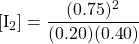Solving,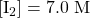The concentration unit is assumed to be molarity. This value for [I2] can be easily verified by substituting 0.75, 0.20, and 7.0 into the expression for Keq and evaluating: you should get 0.40, the numerical value of Keq (and you do).

Test Yourself
Given the following reaction:

H2 + I2 ⇄ 2HI

If the equilibrium [HI] is 0.060 M and the equilibrium [I2] is 0.90 M, what is the equilibrium [H2] if the Keq is 0.40?

0.010 M

In some types of equilibrium problems, square roots, cube roots, or even higher roots need to be analyzed to determine a final answer. Make sure you know how to perform such operations on your calculator; if you do not know, ask your instructor for assistance.

Example 13.3

The following reaction is at equilibrium:

N2 + 3H2 ⇄ 2NH3

The Keq at a particular temperature is 13.7. If the equilibrium [N2] is 1.88 M and the equilibrium [NH3] is 6.62 M, what is the equilibrium [H2]?

Solution
We start by writing the Keq expression from the balanced chemical equation: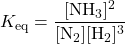Substituting for the known equilibrium concentrations and the Keq, this becomes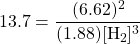Rearranging algebraically and then evaluating the numerical expression, we get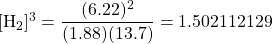To solve for [H2], we need to take the cube root of the equation. Performing this operation, we get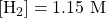You should verify that this is correct using your own calculator to confirm that you know how to do a cube root correctly.

Test Yourself
The following reaction is at equilibrium:

N2 + 3H2 ⇄ 2NH3

The Keq at a particular temperature is 13.7. If the equilibrium [N2] is 0.055 M and the equilibrium [H2] is 1.62 M, what is the equilibrium [NH3]?

1.79 M

The Keq was defined earlier in terms of concentrations. For gas-phase reactions, the Keq can also be defined in terms of the partial pressures of the reactants and products, Pi. For the gas-phase reaction

aA(g) + bB(g) ⇄ cC(g) + dD(g)

the pressure-based equilibrium constant, KP, is defined as follows: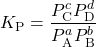where PA is the partial pressure of substance A at equilibrium in atmospheres, and so forth. As with the concentration-based equilibrium constant, the units are omitted when substituting into the expression for KP.

Example 13.4

What is the KP for this reaction, given the equilibrium partial pressures of 0.664 atm for NO2 and 1.09 for N2O4?

2NO2(g) ⇄ N2O4(g)

Solution
Write the KP expression for this reaction: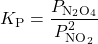Then substitute the equilibrium partial pressures into the expression and evaluate: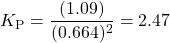Test Yourself
What is the KP for this reaction, given the equilibrium partial pressures of 0.44 atm for H2, 0.22 atm for Cl2, and 2.98 atm for HCl?

H2 + Cl2 ⇄ 2HCl

91.7

There is a simple relationship between Keq (based on concentration units) and KP (based on pressure units):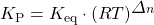where R is the ideal gas law constant (in units of L·atm/mol·K), T is the absolute temperature, and Δn is the change in the number of moles of gas in the balanced chemical equation, defined as ngas,prodsngas,rcts.

Note that this equation implies that if the number of moles of gas are the same in reactants and products, Keq = KP.

Example 13.5

What is the KP at 25°C for this reaction if the Keq is 4.2 × 10−2?

N2(g) + 3H2(g) ⇄ 2NH3(g)

Solution
Before we use the relevant equation, we need to do two things: convert the temperature to kelvins and determine Δn. Converting the temperature is easy: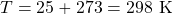To determine the change in the number of moles of gas, take the number of moles of gaseous products and subtract the number of moles of gaseous reactants. There are 2 mol of gas as product and 4 mol of gas of reactant: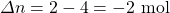Note that Δn is negative. Now we can substitute into our equation, using R = 0.08205 L·atm/mol·K. The units are omitted for clarity: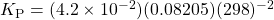Solving,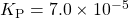Test Yourself
What is the KP at 25°C for this reaction if the Keq is 98.3?

I2(g) ⇄ 2I(g)

2.40 × 103

Finally, we recognize that many chemical reactions involve substances in the solid or liquid phases. For example, a particular chemical reaction is represented as follows:

2NaHCO3(s) ⇄ Na2CO3(s) + CO2(g) + H2O(ℓ)

This chemical equation includes all three phases of matter. This kind of equilibrium is called a heterogeneous equilibrium because there is more than one phase present.

The rule for heterogeneous equilibria is as follows: Do not include the concentrations of pure solids and pure liquids in Keq expressions. Only partial pressures for gas-phase substances or concentrations in solutions are included in the expressions of equilibrium constants. As such, the equilibrium constant expression for this reaction would simply be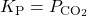because the two solids and one liquid would not appear in the expression.

Key Takeaways

• Every chemical equilibrium can be characterized by an equilibrium constant, known as Keq.
• The Keq and KP expressions are formulated as amounts of products divided by amounts of reactants; each amount (either a concentration or a pressure) is raised to the power of its coefficient in the balanced chemical equation.
• Solids and liquids do not appear in the expression for the equilibrium constant.

Exercises

# Questions

1. Define the law of mass action.
2. What is an equilibrium constant for a chemical reaction? How is it constructed?
3. Write the Keq expression for each reaction.
1. H2 + Cl2 ⇄ 2HCl
2. NO + NO2 ⇄ N2O3
4. Write the Keq expression for each reaction.
1. C2H5OH + NaI ⇄ C2H5I + NaOH
2. PCl3 + Cl2 ⇄ PCl5
5. Write the KP expression for each reaction.
1. 2H2(g) + O2(g) ⇄ 2H2O(g)
2. 2H2O2(g) ⇄ 2H2O(g) + O2(g)
6. Write the KP expression for each reaction.
1. CH4(g) + 2O2(g) ⇄ CO2(g) + 2H2O(g)
2. CH4(g) + 4Cl2(g) ⇄ CCl4(g) + 4HCl(g)
7. The following reaction is at equilibrium:

PBr3 + Br2 ⇄ PBr5

The equilibrium [Br2] and [PBr5] are 2.05 M and 0.55 M, respectively. If the Keq is 1.65, what is the equilibrium [PBr3]?

8. The following reaction is at equilibrium:

CO + Cl2 ⇄ CoCl2

The equilibrium [CO] and [Cl2] are 0.088 M and 0.103 M, respectively. If the Keq is 0.225, what is the equilibrium [COCl2]?

9. The following reaction is at equilibrium:

CH4 + 2Cl2 ⇄ CH2Cl2 + 2HCl

If [CH4] is 0.250 M, [Cl2] is 0.150 M, and [CH2Cl2] is 0.175 M at equilibrium, what is [HCl] at equilibrium if the Keq is 2.30?

10. The following reaction is at equilibrium:

4HBr + O2 ⇄ 2H2O + 2Br2

If [HBr] is 0.100 M, [O2] is 0.250 M, and [H2O] is 0.0500 M at equilibrium, what is [Br2] at equilibrium if the Keq is 0.770?

11. Write the KP expression for the following gas-phase reaction:

4NO2(g) + O2(g) ⇄ 2N2O5(g)

12. Write the KP expression for the following gas-phase reaction:

ClO(g) + O3(g) ⇄ ClO2(g) + O2(g)

13. What is the equilibrium partial pressure of COBr2 if the equilibrium partial pressures of CO and Br2 are 0.666 atm and 0.235 atm and the KP for this equilibrium is 4.08?

CO(g) + Br2(g) ⇄ COBr2(g)

14. What is the equilibrium partial pressure of O3 if the equilibrium partial pressure of O2 is 0.0044 atm and KP for this equilibrium is 0.00755?

3O2(g) ⇄ 2O3(g)

15. Calculate the KP for this reaction at 298 K if the Keq = 1.76 × 10−3.

3O2(g) ⇄ 2O3(g)

16. Calculate the KP for this reaction at 310 K if the Keq = 6.22 × 103.

4NO2(g) + O2(g) ⇄ 2N2O5(g)

17. Calculate the Keq for this reaction if the KP = 5.205 × 10−3 at 660°C.

CO(g) + F2(g) ⇄ COF2(g)

18. Calculate the Keq for this reaction if the KP = 78.3 at 100°C.

4HCl(g) + O2(g) ⇄ 2H2O(g) + 2Cl2(g)

19. Write the correct Keq expression for this reaction.

NaOH(aq) + HCl(aq) ⇄ NaCl(aq) + H2O(ℓ)

20. Write the correct Keq expression for this reaction.

AgNO3(aq) + NaCl(aq) ⇄ AgCl(s) + NaNO3(aq)

21. Write the correct KP expression for this reaction.

CaCO3(s) ⇄ CaO(s) + CO2(g)

22. Write the correct KP expression for this reaction.

C2H2(g) + 2I2(s) ⇄ C2H2I4(g)

1. The relationship between the concentrations of reactants and products of a chemical reaction at equilibrium
1.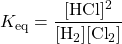2.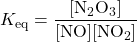1.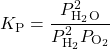2.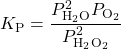1. 0.163 M
1. 0.272 M
1.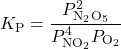1. 0.639 atm
1. 7.20 × 10−5
1. Keq = 3.98 × 10−1
1.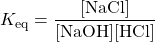1.